# Short & long division worksheets

## Division practice

These division drills worksheets provide practice in short and long division, with emphasis on regrouping (borrowing) and remainders.

We also have division facts and thousands of math worksheets by grade level.

## <!--/*--><![CDATA[/* ><!--*/ <!--td {border: 1px solid #ccc;}br {mso-data-placement:same-cell;}--> /*--><!]]>*/ No remainders

Division, no remainders, 2-digit by1-digit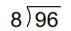Division, no remainders, 3-digit by1-digit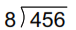Division, no remainders, 4-digit by 1-digit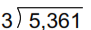Division, no remainders, 5-digit by 1-digit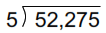Long division, no remainders, 3-digit by 2-digit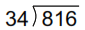Long division, no remainders, 4-digit by 2-digit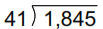Long division, no remainders, 5-digit by 2-digit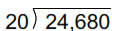Long division, no remainders, 6-digit dividend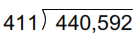Dividing by 10 & 100, no remainders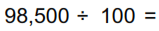Dividing by multiples of 10, no remainders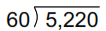Dividing by multiples of 100, no remainders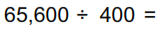## With remainders

Long division, remainders, 2-digit by 1-digit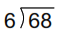Long division, remainders, 3-digit by1-digit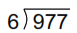Long division, remainders, 4-digit by 1-digit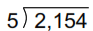Long division, remainders, 5-digit by 1-digit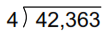Long division, remainders, 3-digit by 2-digit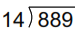Long division, remainders, 4-digit by 2-digit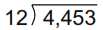Long division, remainders, 5-digit by 2-digit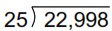Long division, remainders, 6-digit by 3-digit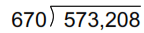Dividing by 10 & 100, remainders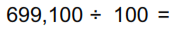Dividing by multiples of 10, remainders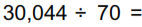Dividing by multiples of 100, remainders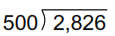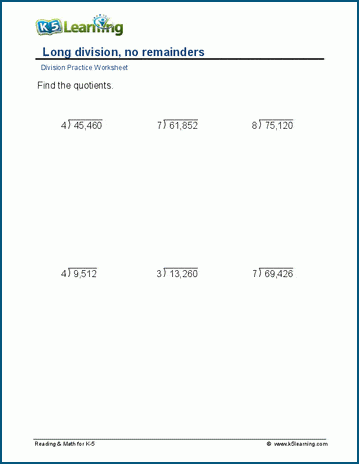Sample division worksheet

What is K5?

K5 Learning offers free worksheets, flashcards and inexpensive workbooks for kids in kindergarten to grade 5. Become a member to access additional content and skip ads.